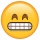Home
IT Knowledge
Inspiration
Languages
EN

# Python - unpack collection

0 points
Created by:Huzaifa-Ball
415

In this article, we would like to show you how to unpack collection in Python.

## 1. Unpack list

In this example, we use universal method to unpack a list but you can use it to extract the values back into variables from any collection.

``````my_list = [1, 2, 3]

x, y, z = my_list

print(x)
print(y)
print(z)``````

Output:

``````1
2
3``````

## 2. Unpack tuple

In this example, we use the same method to unpack a tuple.

``````my_tuple = (1, 2, 3)

x, y, z = my_tuple

print(x)
print(y)
print(z)``````

Output:

``````1
2
3``````